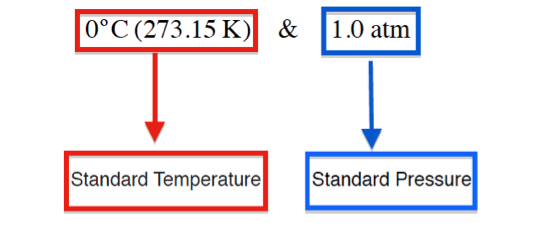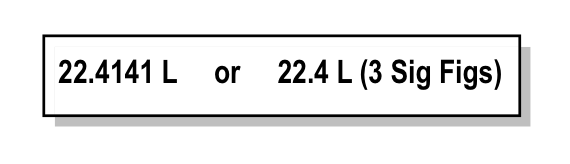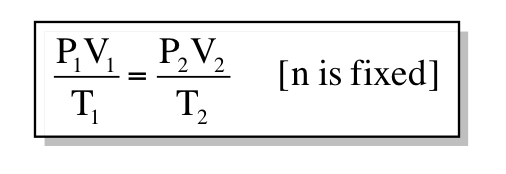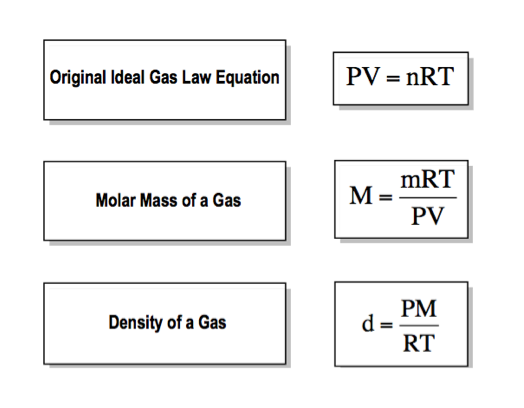Ch.1 - Intro to General Chemistry 2hrs & 53mins 0% complete Worksheet Ch.2 - Atoms & Elements 2hrs & 40mins 0% complete Worksheet Ch.3 - Chemical Reactions 3hrs & 25mins 0% complete Worksheet BONUS: Lab Techniques and Procedures 1hr & 38mins 0% complete Worksheet BONUS: Mathematical Operations and Functions 47mins 0% complete Worksheet Ch.4 - Chemical Quantities & Aqueous Reactions 3hrs & 30mins 0% complete Worksheet Ch.5 - Gases 3hrs & 47mins 0% complete Worksheet Ch.6 - Thermochemistry 2hrs & 28mins 0% complete Worksheet Ch.7 - Quantum Mechanics 2hrs & 35mins 0% complete Worksheet Ch.8 - Periodic Properties of the Elements 1hr & 57mins 0% complete Worksheet Ch.9 - Bonding & Molecular Structure 2hrs & 5mins 0% complete Worksheet Ch.10 - Molecular Shapes & Valence Bond Theory 1hr & 31mins 0% complete Worksheet Ch.11 - Liquids, Solids & Intermolecular Forces 3hrs & 40mins 0% complete Worksheet Ch.12 - Solutions 2hrs & 17mins 0% complete Worksheet Ch.13 - Chemical Kinetics 2hrs & 22mins 0% complete Worksheet Ch.14 - Chemical Equilibrium 2hrs & 26mins 0% complete Worksheet Ch.15 - Acid and Base Equilibrium 4hrs & 42mins 0% complete Worksheet Ch.16 - Aqueous Equilibrium 3hrs & 48mins 0% complete Worksheet Ch. 17 - Chemical Thermodynamics 1hr & 44mins 0% complete Worksheet Ch.18 - Electrochemistry 2hrs & 58mins 0% complete Worksheet Ch.19 - Nuclear Chemistry 1hr & 33mins 0% complete Worksheet Ch.20 - Organic Chemistry 3hrs 0% complete Worksheet Ch.22 - Chemistry of the Nonmetals 2hrs & 1min 0% complete Worksheet Ch.23 - Transition Metals and Coordination Compounds 1hr & 54mins 0% complete Worksheet

# Standard Temperature and Pressure

See all sections

In order to accurately study the effect that changes in pressure, temperature and moles have on volume, chemists will often run their experiments under Standard State conditions

###### Standard Temperature & Pressure

Concept #1: Understanding Standard State Conditions

Transcript

Welcome back, guys! In this new video, we're going to take a look to see what happens to our gas behavior under standard states. We're going to say sometimes chemists want to understand the influences that pressure, volume or temperature have on a particular gas particle. To do this, they do these experiments under what we call standard conditions.
We're going to say that these standard conditions are sometimes referred to as STP. STP just means standard temperature and pressure. We're going to say standard temperature is zero degrees Celsius. which really is 273.15 Kelvin. Standard pressure is one atmosphere.Concept #2: Standard Molar Volume

Transcript

We're going to say under standard state conditions, the volume of one mole of any gas, an ideal gas just means a gas that behaves as though it's by itself. One mole of any gas under STP will then have the same volume. At STP, one mole of carbon dioxide, one mole of nitrogen gas, or one mole of helium gas – all of these gases look different. Some of them are just atoms by themselves. Others are different elements connected together.
Under standard state conditions, under STP, all moles of gases have the same volume. They all have 22.4 liters. We're going to say that this volume is known as our standard molar volume. Remember, under STP conditions, one mole of any type of gas will have that as their volume.

At STP, 1 mole of any ideal gas will occupy exactly 22.4 Liters of volume inside a container.Knowing the meaning of STP allows you to now further manipulate the Ideal Gas Law.

Concept #3: Combined Gas Law

Transcript

Let's look at this example which uses our STP concept. We're going to see a sample of Freon-12 occupies 20.7 liters at 25 degrees Celsius and 947 torrs. Now they're saying what is the volume under standard conditions. Remember, what are they asking me? They're asking me to find the volume. But here they already gave me a volume. This must be my volume 1 and this must be my volume 2. They also gave me a temperature and they gave me a pressure. Here, they’re saying under standard conditions. Standard condition means we have a standard pressure and a standard temperature.
Our P2 would be one 1 atmosphere and our standard temperature would be to 273.15 Kelvin. Since we’re deal with a gas, we write PV equals nRT. Again guys, we're dealing with two sets of data. Remember, what have we done when we have two sets of something, two volumes or two pressures? We’d have to check to see who they're talking about. They're talking about pressure, they’re talking about volume and they're talking about temperature. They're not talking about moles because moles are being held constant, so we ignore it. R is our constant as well so we ignore that too. Everything is not on the same side of the equation sign. Divide everything by T to bring it over to the left. PV over T, since we’re dealing with two sets of data, it’s P1V1 over T1 equals P2V2 over T2.
This creates a whole new type of gas law, which we call the combined gas law. We haven't seen that until now. This is our combined gas law P1V1 over T1 equals P2V2 over T2. This is our combined gas law. We're going to plug in the units that we know into this combined gas law. But first, you have to change the temperature into Kelvin and you have to change those 947 torrs into atmospheres. T1 is 25 degrees Celsius, so all we have to do is add 273.15 which gives us 298.15 Kelvin. Pressure 1 is 947 torrs. We just have to change this to atmospheres. For every 1 atmosphere it's 760 torrs. When we do that, we get our answer as 1.24605 atmospheres. All we have to do now is plug it in and solve for our missing variable. Our P1 is 1.24605 atmospheres. Our volume 1 I told you was 20.7 liters. Our temperature 1 was 298.15
Kelvin, equals.
The pressure 2 was 1 atmosphere. That's really not going to change anything, so we plug it in anyways, 1 atmospheres. V2 is what we're looking for over temperature 2 which is 273.15 Kelvin. What we're going to do here is we’re just going to solve for everything on the left. Do the P1 times the V1 divided by T1. When we do all of that, the left side gives me 0.086511 liters times atmospheres over K. This still equals my 1 atmosphere times V2 divided by 273.15 Kelvin. Multiply both sides now by the 273.15 Kelvin. Kelvins cancel out, so then that’s going to give me 23.6305 liters times atmospheres still equals 1 atmosphere times V2. Divide both sides by the 1 atmosphere, which doesn't do anything. All it does is it helps get rid of these atmospheres in the units. So then V2 equals, we're going to just do 3 sig figs here. It's 23.6 liters. That would be the answer for our volume 2.
Again guys, remember even though we started out with the ideal gas law, because we have two sets of data again, we have to manipulate the ideal gas law. Doing that helped us isolate our answer here for V2. Now that you’ve seen this and now that you understand what STP is, I want you guys to attempt the next question.Practice: What volume will 5.72 x 1025 atoms of argon gas occupy at STP?

###### Gas Law Applications

Concept #4: Using the Ideal Gas Law to find density or molar mass

Transcript

We're going to say that we're used to seeing the ideal gas law as PV equals nRT. But we should realize that we can manipulate this equation further in order to find the molar mass of the gas, or the density of a gas. Here, to find the molar mass of a gas, we change it. This capital M is not molarity, it's molar mass. Remember, the units for molar mass or molecular mass are grams per mole. We’re going to say the molar mass of a gas is equal to mRT over PV. We're going to say m represents the mass of the gas in grams, and then all the other variables stay the same. R is still our gas constant. Temperature is still in Kelvin. P is still in atmospheres and volume is still in liters.
If we want to find the density of a gas, we could say the density of a gas is equal to pressure times the molar mass divided by RT. This can become our equation. We could also further manipulate if we wanted the second equation. We could have combined mass and volume together to give us one thing. Remember, mass over volume helps us find density. We could have manipulated this further by saying molar mass of a gas is equal to density times RT over P. We could have used this as well. But then again, if we have a question dealing with the density of a gas, we know we could use this one and we could just manipulate it.
Even though this is a potential equation, we can get to it by just manipulating this third one. I’d recommend just remembering these three. If you're dealing with a question that gives you density and wants the molecular weight, you just use the third one. Manipulate it to find your answer.

The Ideal Gas Law can be further manipulated into new equations. These new equations can help us find the density or molar mass of a gas.When calculating the density of a gas it is essentially to also recall the formula of that gas in order to find the molar mass, M.

Practice: What is the density of chlorine gas at STP?

Remember that units for molar mass are grams of compound divided by the moles of that compound.

Practice: An unknown gas sample weighs 3.12 g. If it has a volume of 0.206 µL when the temperature is 45oC and the pressure is 957 torr. What is its molar mass?